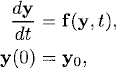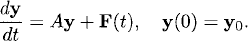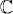# SYSTEMS OF DIFFERENTIAL EQUATIONS

In this chapter we motivate, by means of a simple observation, the generalization to systems of equations of what we have learned for scalar differential equations.

Consider the initial value problem for a system of differential equations

(6.1)where y is an N-component vector and f is an N-component vector-valued function:The simplest systems are linear systems with constant coefficients:

(6.2)Here A is a constant N x N-matrix and F has N components.

Let us assume that A has a complete set of eigenvectors; that is, there exists a transformation S that transforms A into a diagonal matrix λ:Exercise 6.1 The exponential of a matrix ANxN is defined via the Taylor series,(a) Show that exp(A) is well defined (i.e., the series is always convergent). ...

Get Introduction to Numerical Methods for Time Dependent Differential Equations now with the O’Reilly learning platform.

O’Reilly members experience live online training, plus books, videos, and digital content from nearly 200 publishers.